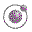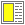Expressions, Types, and Functions - slide 28 : 46

Vectors
Vectors in Scheme are heterogeneous array-like data structures of a fixed size
• Vectors are denoted in a similar way as list

• Example: #(0 a (1 2 3))

• Vectors must be quoted in the same way as list when their external representations are used directly

• The function vector is similar to the function list

• There are functions that convert a vector to a list and vice versa

• vector->list

• list->vector

The main difference between lists and vectors is the mode of access and the mode of construction